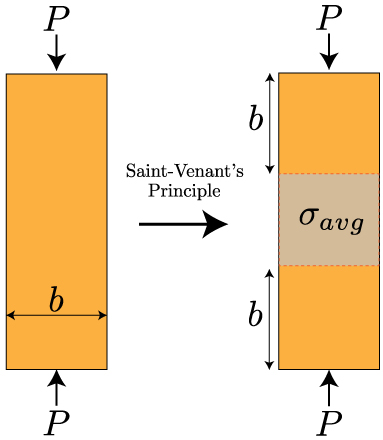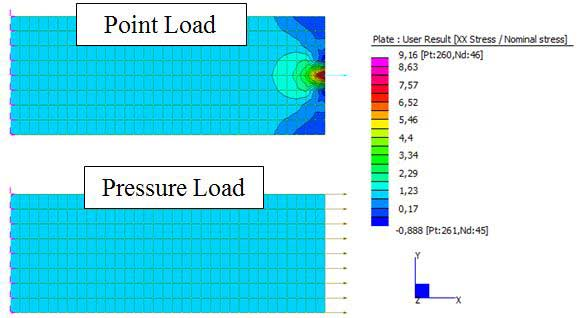CONSELF | Dealing with stress singularities and concentrations
9958# Dealing with stress singularities and concentrations## 06 Feb Dealing with stress singularities and concentrations

FEM (Finite Element Method) analysis are becoming more and more popular with the development of modern instruments of analysis. Nevertheless currently a higher number of people are struggling with simulations, in particular are trying to solve the stress singularities and concentrations problem. These two problems are fundamental to understand the quality of the simulation and to obtain some design guidelines out of them.

INTRODUCTION

FEM analysis, as you all may know already, allows to find stress and displacement inside a continuum domain. To perform a FEM analysis the domain must be divided into smaller and elementary elements. This procedure, know as discretization, is fundamental to obtain accurate results: as the elementary elements dimension becomes smaller generally the solution converge towards the exact problem solution.

This is not always true and this post illustrates some problems often users may encounter.

STRESS SINGULARITIES

A stress singularity is defined as a point where the stress does not converge towards a specific value when the basic elements dimension is reduced. In these points the stress level measured with a FEM analysis keeps increasing while the mesh is refined up to … an infinite value!When a load is applied to a simple plate, it is possible to define a certain distance b where local effects are dominant. Out of this zone, according to Saint-Venant’s Principle, stresses are no more affected by the method in which the load is applied 

Geometry highly affect the presence of these type of occurrences: appliance of a point load, sharp re-entrant corners, corners of bodies in contact and point restraints are the most common situations which should be avoided. Keep in mind that these stress singularities does not affect the quality of the simulation: according to the St. Veneant’s Principle it is possible to define a distance away from the singularity where stress results are going to be fine and representative of the physical reality.

Load application may then cause singularities, in particular if we are considering point load forces (i.e. force applied to a single node). The following image clearly shows the influence of the load type to the simulation results in terms of singularities.Another typical situation where singularities occur are sharp re-entrant corners. These are points where the geometry shows an angle lower than 180°. In the example showed we have a 90° angles. Once again, even if we note the stress singularity at the corner, it is possible to neglect it according to the above mentioned principle. In a real geometry, since no corner is actually perfect, a small fillet radius is present. This means that in reality infinite stress obviously can’t occur. We generally see stress concentration instead.Example of a hole inside a plate. Stress concentration occur at the two side of the hole, where loads lines tend to gets closer and closer

STRESS CONCENTRATION

Let’s now get into the details of a similar phenomena: stress concentration. What now happens is something similar to a singularity point, but this time the stress converge towards a finite value the more we refine our mesh. This sort of behavior is due to the presence of a geometrical feature that somehow deflect the load pattern inside of our body. For example a hole in a plate.

In the picture above, showing stress concentration around a small circular hole, we see that on the two sides of such a hole stress field tend to become more intense. This is, obviously, due to the geometry we are analyzing and FEM analysis is able to capture this behavior (as long as the mesh is refined enough!). In general, for such type of plate, it is possible to define a concentration factor, ratio between the maximum stress and the average stress in the undisturbed plate, named nominal stress. In literature is possible to find out many relations to find out the stress factor as in  and .

CONCLUSIONS

In FEM (Finite Element Method) the user has the great responsibility of detecting singularities and distinguish between them and concentration points. Obviously a mesh sensitivity study is mandatory to find out details about the case we are performing. In general it is possible to say that the main difference between the two behaviors is that singularities tends to have infinite stress as long as we refine the mesh, whilst concentrations tends to assume a finite value.

Did you like this post? Try also these ones:

If you want now you can start taking advantage of CONSELF FEA/FEM on the cloud. Register here: https://conself.com/my-account/ !

__________________

REFERENCES

 Saint-Venant’s Principle, Wikipedia, available at: http://es.wikipedia.org/wiki/Principio_de_Saint-Venant

 Young, W., Budynas, R., (2001), Roark’s Formulas for Stress and Strain, Chapter 17 – Stress Concentrations, McGraw-Hill.

 Pilkey, D., Pilkey, W., (2008), Peterson’s Stress Concentration Factors, Wiley; 3 edition.